## Excel Formula: Assign points based on late time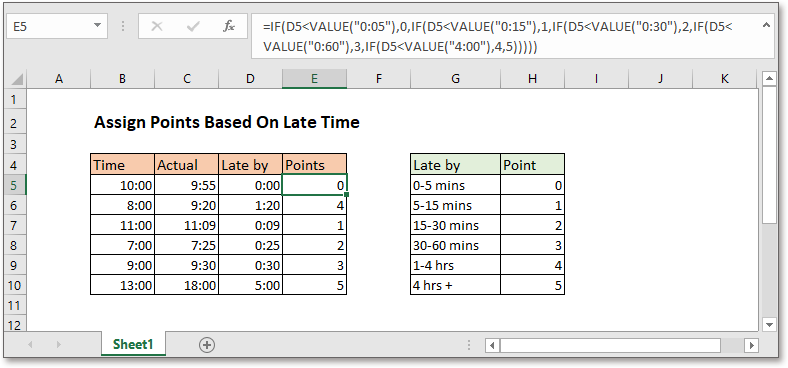This tutorial provides a clear guide on how to use the IF function to assign points on late time in Excel.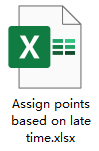Generic formula:

 IF(late_by_time

For better readability, here use line breaks to display the long formula.

Arguments

 Late_by_time: the late time.

Return value

This formula returns the assign pointes based on late time.

How this formula work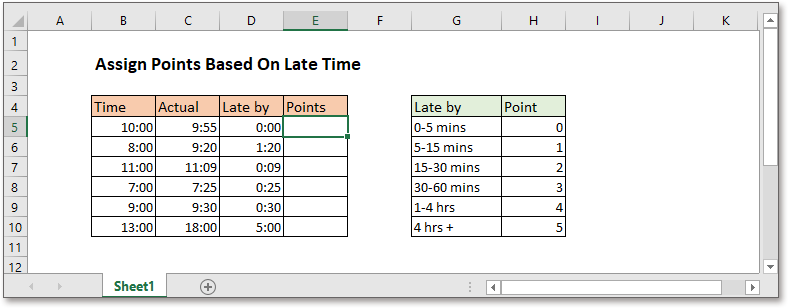Take instance, here is a list of point rules and time records, please use below formula:

 =IF(D5

Press Enter key, the related point has been displayed.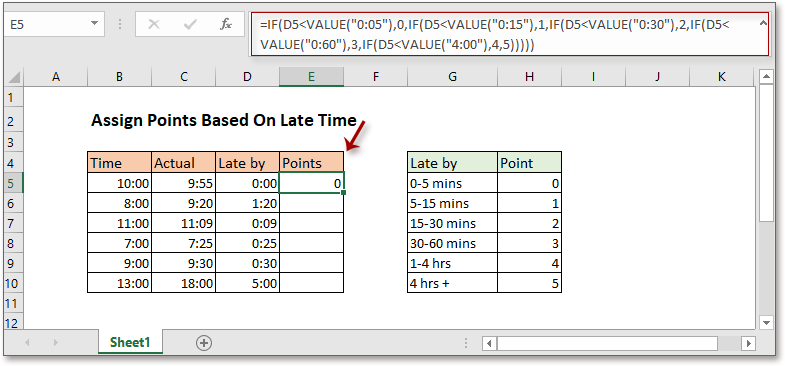Then drag fill handle over the cells to apply this formula.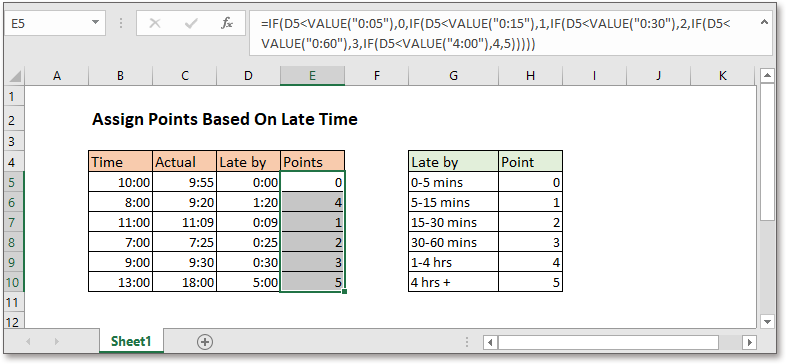Explanation

IF function is used to test for specified conditions, then returns the corresponding values. Here, explain the formula with 5 parts:

IF(late_by_time<VALUE("0:05"),0, if the late time is less than 5 minutes, it returns 0.

IF(late_by_time <VALUE("0:15"),1, if the late time is less than 15 minutes but greater than or equal to 5 minutes, it returns 1.

IF(late_by_time <VALUE("0:30"),2, if the late time is less than 30 minutes but greater than or equal to 15 minutes, it returns 2.

IF(late_by_time <VALUE("0:60"),3, if the late time is less than 60 minutes but greater than or equal to 30 minutes, it returns 3.

IF(late_by_time <VALUE("4:00"),4, if the late time is less than 4 hours but greater than or equal to 60 minutes, it returns 4.

5))))) if the late time is greater than or equal to 4 hours, it returns 5.

Value function is used to convert text to number. Here it converts the text time to number.

Note

To calculate the late by time, please use this formula:

 =IF(C5-B5<0,0,C5-B5)

If the calculation results are not in the time format, you can right click the calculation results and select Format Cells from the context menu. Then in the Format Cells dialog, enable Time in the Category list box, click to select the time format of 13:30, and click OK.